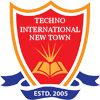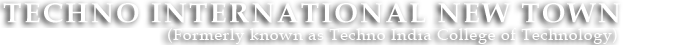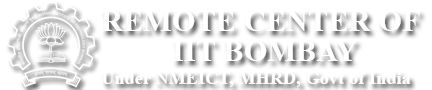#+91 9674112076/2079
••••# Basic Electronics Engineering Lab

BASIC ELECTRONICS ENGINEERING LABORATORY-I

Code : ES 191(EC)

List of Experiments:

1. Familiarization with passive and active electronic components such as Resistor, Inductor, Capacitor, Diode, Transistor (BJT) etc.
2. Familiarization with equipment like DC power supply, multimeter, CRO, Function generator etc.
3. Study of V-I characteristics of Junction diode.
4. Study of V-I characteristics of Zener diodes.
5. Study of Half wave rectifier with regulation efficiency and ripple factor.
6. Study of Bridge rectifier with regulation efficiency and ripple factor.
7. Study of input characteristics and output characteristics of BJT in CE configuration.

BASIC ELECTRONICS ENGINEERING LABORATORY-II

Code: ES 291(EC)

List of Experiments:

1. Study of input characteristics and output characteristics of BJT in CB configuration.
2. Study of transfer characteristics and output characteristics of Junction Field Effect Transistor (JFET).
3. Study of transfer characteristics and output characteristics of Metal Oxide Semiconductor Field Effect Transistor (MOSFET).
4. Determination of input-offset voltage, input bias current and Slew rate of OPAMPs.
5. Determination of Common-mode Rejection ratio, Bandwidth and Off-set null of OPAMPs.
6. Study of different OPAMP circuits like Inverting and Non-inverting amplifier, Adder, Integrator, Differentiator etc.
7. Study of Logic Gates and realization of Boolean functions using Logic Gates.